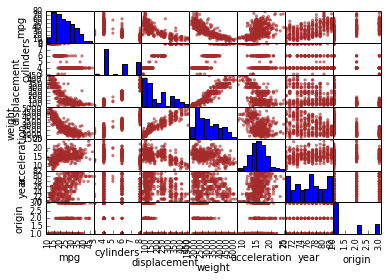Chapter 2: Basic Operations¶

Vectors, Data, Matrices and Subsetting¶

In :
from __future__ import division
import pandas as pd
import numpy as np
import matplotlib.pyplot as plt
%matplotlib inline
In :
x = np.array([2,7,5])    # explicit vector creation
x
Out:
array([2, 7, 5])
In :
y = np.arange(4, 13, 3)  # vector creation from a sequence (start, stop, step)
y
Out:
array([ 4,  7, 10])
In :
x + y    # vectors can be added
Out:
array([ 6, 14, 15])
In :
x / y    # divided
Out:
array([ 0.5,  1. ,  0.5])
In :
x ** y    # exponentiated
Out:
array([     16,  823543, 9765625])
In :
x    # vector elements can be selected by position
Out:
7
In :
x[1:3]  # multiple elements can be selected using slices
Out:
array([7, 5])
In :
x[-2]  # elements can be specified as offset from end
Out:
7
In :
x[np.array([0,1])]  # elements can be specified as an array
Out:
array([2, 7])
In :
Z = np.matrix(np.arange(1,13)).reshape((4, 3))
Z                 # note: R arranges the elements column-wise
Out:
matrix([[ 1,  2,  3],
[ 4,  5,  6],
[ 7,  8,  9],
[10, 11, 12]])
In :
Z[2:4, 1:3]    # R is 1-based and includes ending index, Python is 0 based and does not.
Out:
matrix([[ 8,  9],
[11, 12]])
In :
Z[:, 1:3]    # column slice
Out:
matrix([[ 2,  3],
[ 5,  6],
[ 8,  9],
[11, 12]])
In :
Z.shape
Out:
(4, 3)

Generating Random Data, Graphics¶

In :
x = np.random.uniform(0.0, 1.0, 50)
x
Out:
array([ 0.65268288,  0.55438063,  0.12332376,  0.73656161,  0.172588  ,
0.6952661 ,  0.36758793,  0.42034067,  0.54835434,  0.85493252,
0.36666796,  0.14029571,  0.16910043,  0.18956811,  0.77016189,
0.1914512 ,  0.48202498,  0.98936099,  0.43339457,  0.3690124 ,
0.91976653,  0.42354589,  0.23428098,  0.24846295,  0.20409963,
0.71024602,  0.57361799,  0.12510961,  0.70550836,  0.62120022,
0.7089459 ,  0.67670746,  0.39836928,  0.17109468,  0.57367169,
0.60584339,  0.72445482,  0.67991423,  0.94658844,  0.91068048,
0.65489981,  0.05985463,  0.56797862,  0.67604148,  0.989085  ,
0.79619792,  0.46406902,  0.91227664,  0.38043679,  0.04246386])
In :
y = np.random.normal(0.0, 1.0, 50)
y
Out:
array([ -5.95648785e-01,   1.54445571e+00,   1.35312925e+00,
1.88123098e+00,  -3.80353324e-01,  -9.67551972e-04,
5.97422145e-01,   3.76114342e-02,  -1.33779968e+00,
-5.95407697e-01,   1.27206236e+00,  -1.70114410e+00,
-1.84147571e+00,   7.16611666e-01,   2.33006825e+00,
-6.56709283e-01,   8.26951067e-01,   4.64854930e-01,
8.79290232e-01,  -7.42861574e-02,   4.88797828e-01,
1.50860241e+00,   1.07467313e+00,  -7.99843607e-01,
6.81719603e-02,   5.06424430e-01,   2.15770333e-01,
1.67843398e+00,  -5.01086886e-01,   1.51407152e-01,
-3.30648417e-02,  -8.50683506e-01,  -1.56531920e+00,
-6.43438018e-01,  -9.55514605e-01,   1.51696803e-01,
-9.87417666e-01,  -4.44820480e-01,   1.21902188e-01,
-5.20474443e-01,   8.31544982e-01,  -6.75527626e-01,
1.00537660e+00,   4.87948062e-01,   3.43123002e-01,
3.95408496e-01,   4.27046930e-01,   3.69945068e-01,
1.36728064e+00,  -3.15495180e-01])
In :
fig, ax = plt.subplots()
plt.scatter(x, y)
Out:
<matplotlib.collections.PathCollection at 0x420b410>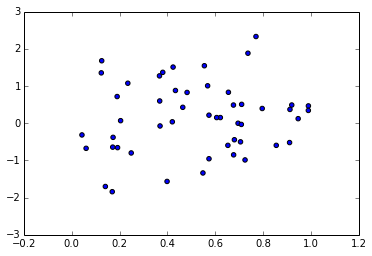In :
fig, ax = plt.subplots()
plt.xlabel("Random Uniform")
plt.ylabel("Random Normal")
plt.scatter(x, y, marker='o', color='red')  # plot customizations
Out:
<matplotlib.collections.PathCollection at 0x4241f50>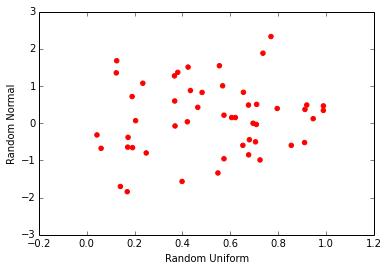In :
plt.subplot(121)    # parameter indicates 1 rows, 2 col, first figure
plt.scatter(x, y)
plt.subplot(122)
plt.hist(y)
Out:
(array([ 3.,  1.,  9.,  5.,  9.,  9.,  6.,  3.,  4.,  1.]),
array([-1.84147571, -1.42432131, -1.00716692, -0.59001252, -0.17285812,
0.24429627,  0.66145067,  1.07860506,  1.49575946,  1.91291385,
2.33006825]),
<a list of 10 Patch objects>)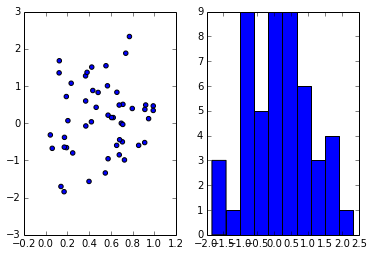In :
# data comes from ISL book site: http://www-bcf.usc.edu/~gareth/ISL/data.html
auto_df.columns     # column names
Out:
Index([u'mpg', u'cylinders', u'displacement', u'horsepower', u'weight', u'acceleration', u'year', u'origin', u'name'], dtype='object')
In :
auto_df.shape    # number of rows, number of columns
Out:
(397, 9)
In :
type(auto_df)
Out:
pandas.core.frame.DataFrame
In :
auto_df.describe()  # equivalent of R's DataFrame.summary()
Out:
mpg cylinders displacement weight acceleration year origin
count 397.000000 397.000000 397.000000 397.000000 397.000000 397.000000 397.000000
mean 23.515869 5.458438 193.532746 2970.261965 15.555668 75.994962 1.574307
std 7.825804 1.701577 104.379583 847.904119 2.749995 3.690005 0.802549
min 9.000000 3.000000 68.000000 1613.000000 8.000000 70.000000 1.000000
25% 17.500000 4.000000 104.000000 2223.000000 13.800000 73.000000 1.000000
50% 23.000000 4.000000 146.000000 2800.000000 15.500000 76.000000 1.000000
75% 29.000000 8.000000 262.000000 3609.000000 17.100000 79.000000 2.000000
max 46.600000 8.000000 455.000000 5140.000000 24.800000 82.000000 3.000000

8 rows × 7 columns

In :
plt.ylabel("MPG")
auto_df.plot(x="cylinders", y="mpg", style='o')
Out:
<matplotlib.axes.AxesSubplot at 0x46ef650>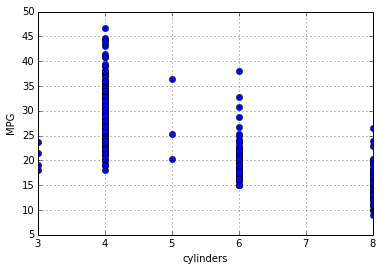In :
auto_df.boxplot(column="mpg", by="cylinders") # MPG distribution by number of cylinders
Out:
<matplotlib.axes.AxesSubplot at 0x499f510>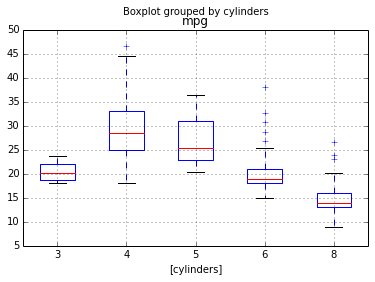In :
# similar to R pairs, shows correlation scatter plots between columns and distribution for each
# column along the diagonal.
# The R version that uses formulas does not seem to have a Python equivalent (and doesn't seem
# to be very useful for exploratory analysis IMO).
axes = pd.tools.plotting.scatter_matrix(auto_df, color="brown")